## A sphere made of plastic has a density of 1.36 g/cm3 and a radius of 8.50 cm. It falls through air of density 1.20 kg/m3 and has a drag coef

Question

A sphere made of plastic has a density of 1.36 g/cm3 and a radius of 8.50 cm. It falls through air of density 1.20 kg/m3 and has a drag coefficient of 0.500. What is its terminal speed (in m/s)? From what height (in m) would the sphere have to be dropped to reach this speed if it fell without air resistance?

in progress 0
3 weeks 2021-08-30T15:49:09+00:00 1 Answers 0 views 0

The answers to the questions are

Part 1

The terminal speed of the sphere (in m/s) is 44.8973 m/s.

Part 2

The sphere has to be dropped from a height of 102.74 m to reach the speed of 44.8973 m/s if it fell without air resistance.

(102.74 m).

Explanation:

Part 1

Terminal velocity is given by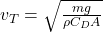Where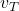= Terminal velocity

m = Mass of the sphere = 3.498 kg

g = Acceleration due to gravity = 9.81 m/s²

ρ = Density of the air = 1.20 kg/m³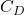= Drag coefficient = 0.500

A = Object cross sectional area = 0.022698 m²

The mass of the sphere is given by

Density =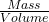where volume of the sphere is given by

Volume =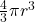Where

r = radius of the sphere = 8.50 cm = ‪0.085‬ m

Therefore the volume V = 2572.44 cm³ = 2.57×10⁻³ m³

The mass then becomes, Mass = Density × Volume  = 1.36×2572.44 = 3498.5184 g = 3.498 kg

The mass, m of the sphere = 3.498 kg

The cross sectional area of the sphere is given by A = π×r² = 0.022698 m²

Therefore the terminal velocity becomes=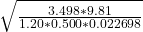= 44.8973 m/s.

Part 2

To reach the speed of 44.8973 m/s in free fall, we have

v² = u² + 2·g·h where

u = initial velocity = 0 m/s

h = Height from which the sphere needs to be dropped

v = final sphere velocity without air resistance

Therefore v² = 2·g·h ⇒ 44.8973² = 2×9.81×h

h = 44.8973²÷(2×9.81)

= 102.74 m.